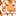Announcements
MAINTENANCE ALERT: Dec. 12th starting 9 AM CET. Community will be read-only. GET DETAILS
cancel
Showing results for
Did you mean:Creator

## Aggr Expression help.

I have the below exoression in bar chart and my dimension is year & Qtr.

I need to get the sum on Distinct Code.How can I do that ?

Sum(Aggr(sum(if([Type]='Direct Lease',SQF_Committed)) <=0,

sum(if([Type]='Direct Lease',SQF_Committed_Planned),

sum(if([Type]='Direct Lease',SQF_Committed))),CodeKey))

I tried the bwlow exoression,but it dosnt work.

Sum(Aggr(sum(if([Type]='Direct Lease',SQF_Committed)) <=0,

sum(if([Type]='Direct Lease',SQF_Committed_Planned),

sum(if([Type]='Direct Lease',SQF_Committed))),distinct CodeKey))

Thanks much.

1 Solution

Accepted SolutionsMVP

Try this

=Sum({<[Type] = {'Direct Lease'}>}Aggr (Avg({<[Type] = {'Direct Lease'}>} SF), Code))

or

=Sum(Aggr (Avg({<[Type] = {'Direct Lease'}>} SF), Code))

8 RepliesMVP

Not sure what you trying to do, but are you missing an if statement?

Sum(Aggr(

If(Sum({<Type = {'Direct Lease'}>} SQF_Committed) <= 0,

Sum({<Type = {'Direct Lease'}>} SQF_Committed_Planned),

Sum({<Type = {'Direct Lease'}>} SQF_Committed))

, CodeKey))

or

Sum(Aggr(

If(Sum({<Type = {'Direct Lease'}>} SQF_Committed) <= 0,

Sum({<Type = {'Direct Lease'}>} SQF_Committed_Planned),

Sum({<Type = {'Direct Lease'}>} SQF_Committed))

, CodeKey, Year, Qtr))Creator
Author

Thank you Sunny.

Sum(Aggr(sum(if([Type]='Direct Lease',SQF_Committed)) <=0,

sum(if([Type]='Direct Lease',SQF_Committed_Planned),

sum(if([Type]='Direct Lease',SQF_Committed))),CodeKey))

This expression works fine but when I have same CodeKey twice its double counting the SQF.

I want to get the sum of SQF on distinct CodeKey.Can I do that ?

@sunnyt  stalwar1MVP

Would you be able to share a sample to show the issue?Creator
Author

PFA sample file and QVW.

I need to get the sum of SF on distinct Code instead of 1000 it should return 500.Is it doable ?

Thanks much.MVP

Try this

=Sum({<[Type] = {'Direct Lease'}>}Aggr (Avg({<[Type] = {'Direct Lease'}>} SF), Code))

or

=Sum(Aggr (Avg({<[Type] = {'Direct Lease'}>} SF), Code))Creator
Author

Awesome.You are my saving graceCreator
Author

Hello there...

can I put Max on this expression to get the Maximun SF among all the code.MVP

I believe so... have you tried it isn't working?Community Browser